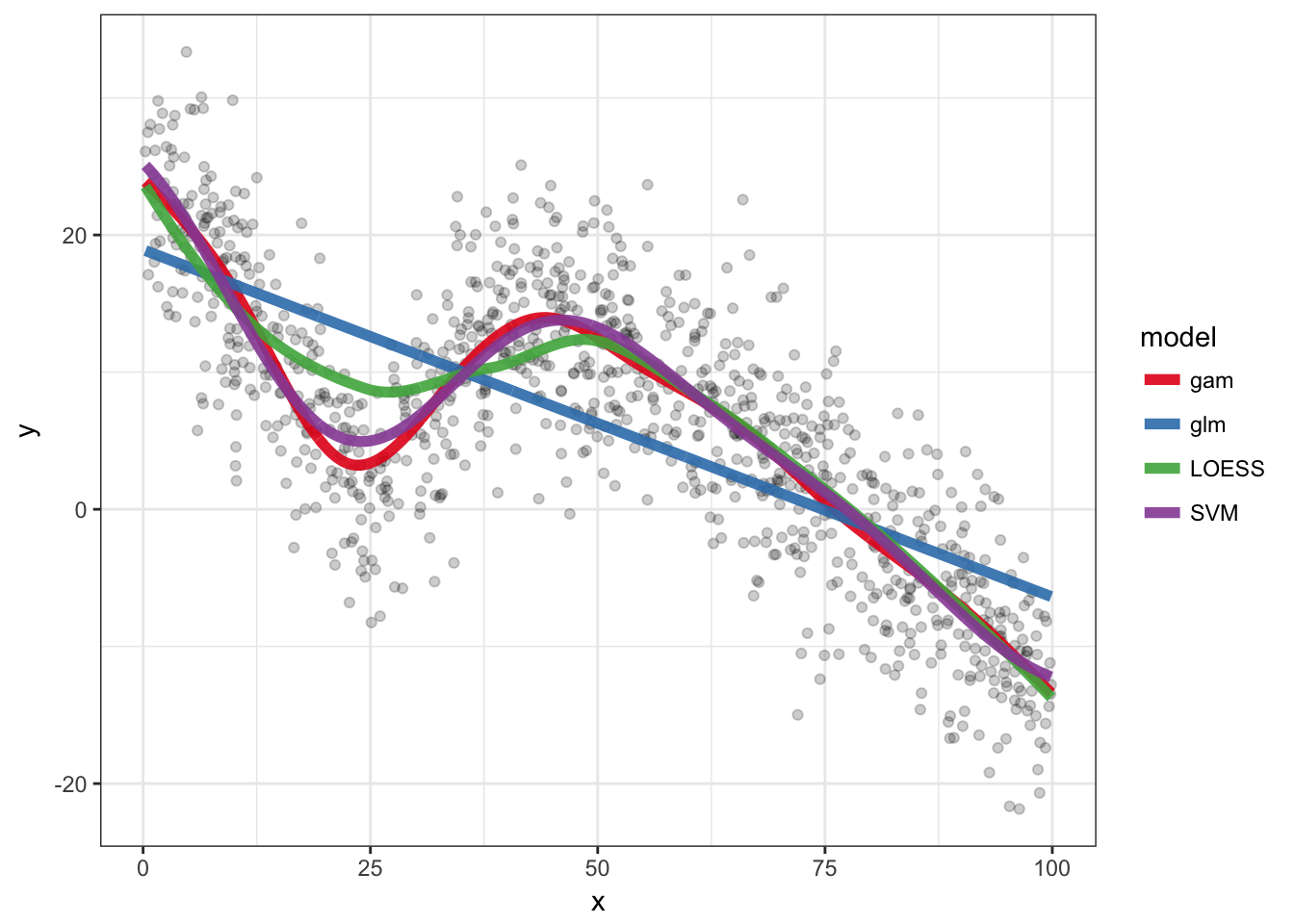# Regression sandbox

library(ggplot2)
library(broom)
library(magrittr)
library(dplyr)
library(knitr)

## Logistic regression

### One way or another

If we have two binary variables and we want to see if they are associated we could use a logistic regression. How do we decide which variable to be the predictor and which variable to observed variable ?

In theory there shouldn’t be any differences but let’s check with a dummy example:

df = data.frame(x = sample(c(FALSE, TRUE), 100, TRUE))
df$y = df$x
df$y[1:70] = sample(c(FALSE, TRUE), 70, TRUE) glm(y ~ x, data = df, family = binomial()) %>% tidy %>% kable term estimate std.error statistic p.value (Intercept) -0.2336149 0.3070802 -0.7607617 0.4467994 xTRUE 1.1745982 0.4256623 2.7594604 0.0057897 glm(x ~ y, data = df, family = binomial()) %>% tidy %>% kable term estimate std.error statistic p.value (Intercept) -0.4054651 0.3227486 -1.256288 0.2090117 yTRUE 1.1745982 0.4256624 2.759460 0.0057897 df$z = runif(100)
glm(y ~ x + z, data = df, family = binomial()) %>%
tidy %>% kable
term estimate std.error statistic p.value
(Intercept) -0.5930811 0.5436028 -1.0910191 0.2752645
xTRUE 1.2012521 0.4293265 2.7979921 0.0051421
z 0.6601875 0.8199692 0.8051369 0.4207407
glm(x ~ y + z, data = df, family = binomial()) %>%
tidy %>% kable
term estimate std.error statistic p.value
(Intercept) -0.1246648 0.5141828 -0.2424523 0.8084297
yTRUE 1.1996170 0.4291206 2.7955243 0.0051816
z -0.5586854 0.8023449 -0.6963157 0.4862311

Adding another predictor doesn’t change the estimates either.

### Interpretation

Just to make sure I understand the estimates correctly. It represents the log odds ratio change for each “unit” of the predictor. In the case of a binary variable, the log odds ratio between the two groups.

glm(y ~ x, data = df, family = binomial()) %>% tidy %>%
kable
term estimate std.error statistic p.value
(Intercept) -0.2336149 0.3070802 -0.7607617 0.4467994
xTRUE 1.1745982 0.4256623 2.7594604 0.0057897
odds.y.ifx = mean(subset(df, x)$y)/mean(!subset(df, x)$y)
odds.y.ifnotx = mean(subset(df, !x)$y)/mean(!subset(df, !x)$y)
log(odds.y.ifx/odds.y.ifnotx)
##  1.174598

### Extreme cases

How efficient is the logistic regression when there is an imbalance between different types of observations ? For example if just a few genomic regions overlap an interesting annotation and I want to test is the overlap is significant.

Let’s look at the worst cases when there are only 1 observation for a particular class.

df = data.frame(y = sample(c(FALSE, TRUE), 100, TRUE))
df$x = 1:nrow(df) %in% sample.int(nrow(df), 1) glm(y ~ x, data = df, family = binomial()) %>% tidy %>% kable term estimate std.error statistic p.value (Intercept) 0.1010961 0.2012644 0.5023050 0.6154530 xTRUE 15.4649721 1455.3975462 0.0106259 0.9915219 Although the significance is low, the estimate seems quite high. I’ll repeat this process a bunch of time and with different number of supporting observations to have an idea of the distribution. ext.df = lapply(1:500, function(ii) { res = lapply(1:10, function(ssi) { df$x = 1:nrow(df) %in% sample.int(nrow(df),
ssi)
glm(y ~ x, data = df, family = binomial()) %>%
tidy %>% mutate(rep = ii, ss = ssi)
})
do.call(rbind, res)
})
ext.df = do.call(rbind, ext.df)
ext.df %>% filter(term == "xTRUE") %>% ggplot(aes(x = estimate)) +
geom_density(fill = "grey50") + facet_grid(ss ~
., scales = "free") + theme_bw()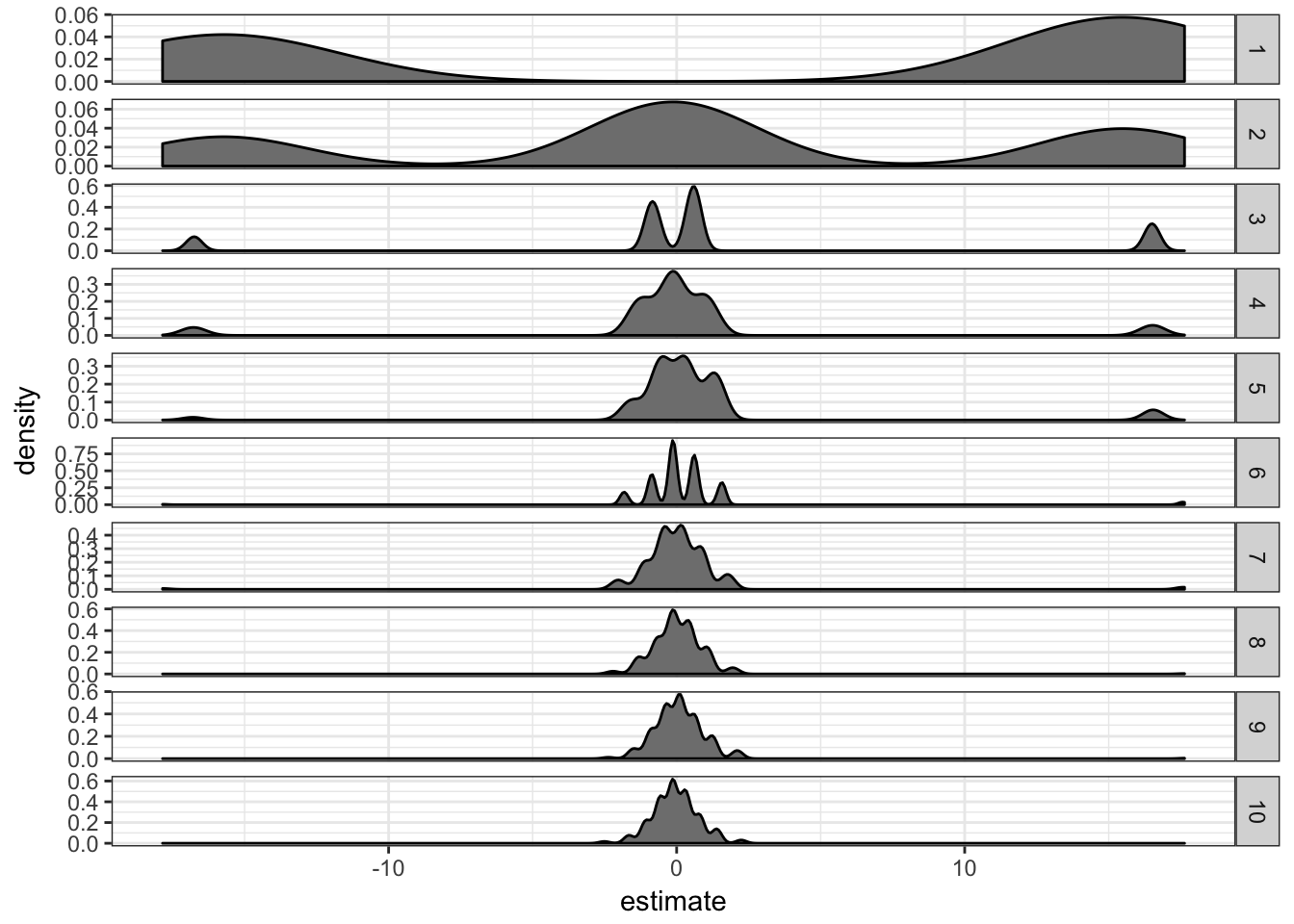It seems like the estimate “inflation” is problematic mostly when there are only 1 or 2 supporting observations. If there are more than 5 supporting observations the estimate is correctly centered in 0.

This problem is in fact called the problem of separation. There are two approaches to deal with it:

1. Firth logistic regression.
2. Exact logistic regression.

The rms package from Frank Harell. It implements a penalized maximum likelihood estimation of the model coefficients through the lrm function which has a penalty= parameter.

library(rms)
extrms.df = lapply(1:200, function(ii) {
res = lapply(1:10, function(ssi) {
res = lapply(c(1, 3, 5), function(pen) {
df$x = 1:nrow(df) %in% sample.int(nrow(df), ssi) cc = lrm(y ~ x, data = df, penalty = pen)$coefficient
data.frame(term = names(cc), estimate = cc,
rep = ii, ss = ssi, penalty = pen,
stringsAsFactors = FALSE)
})
do.call(rbind, res)
})
do.call(rbind, res)
})
extrms.df = do.call(rbind, extrms.df)
extrms.df %>% filter(term == "x") %>% ggplot(aes(x = estimate)) +
geom_density(fill = "grey50") + facet_grid(ss ~
penalty, scales = "free") + theme_bw()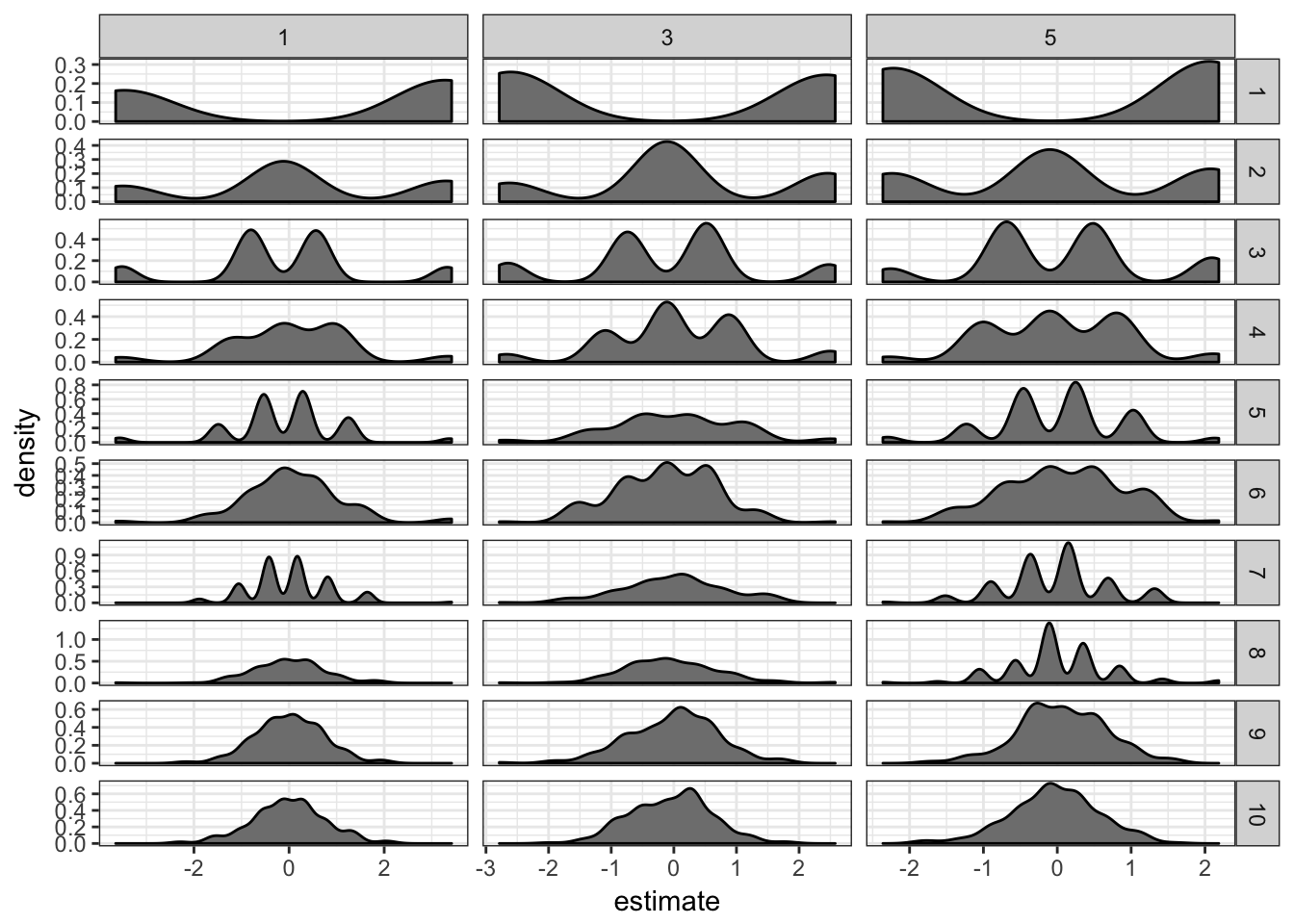It definitely helps: the estimates are now much closer to 0. I don’t see much difference between penalties 1, 3 or 5.

The logistf package. It implements Firth’s bias reduction method with its logistf function.

library(logistf)
extstf.df = lapply(1:200, function(ii) {
res = lapply(1:10, function(ssi) {
df$x = 1:nrow(df) %in% sample.int(nrow(df), ssi) cc = logistf(y ~ x, data = df)$coefficient
data.frame(term = names(cc), estimate = cc,
rep = ii, ss = ssi, stringsAsFactors = FALSE)
})
do.call(rbind, res)
})
extstf.df = do.call(rbind, extstf.df)
extstf.df %>% filter(term == "xTRUE") %>% ggplot(aes(x = estimate)) +
geom_density(fill = "grey50") + facet_grid(ss ~
., scales = "free") + theme_bw()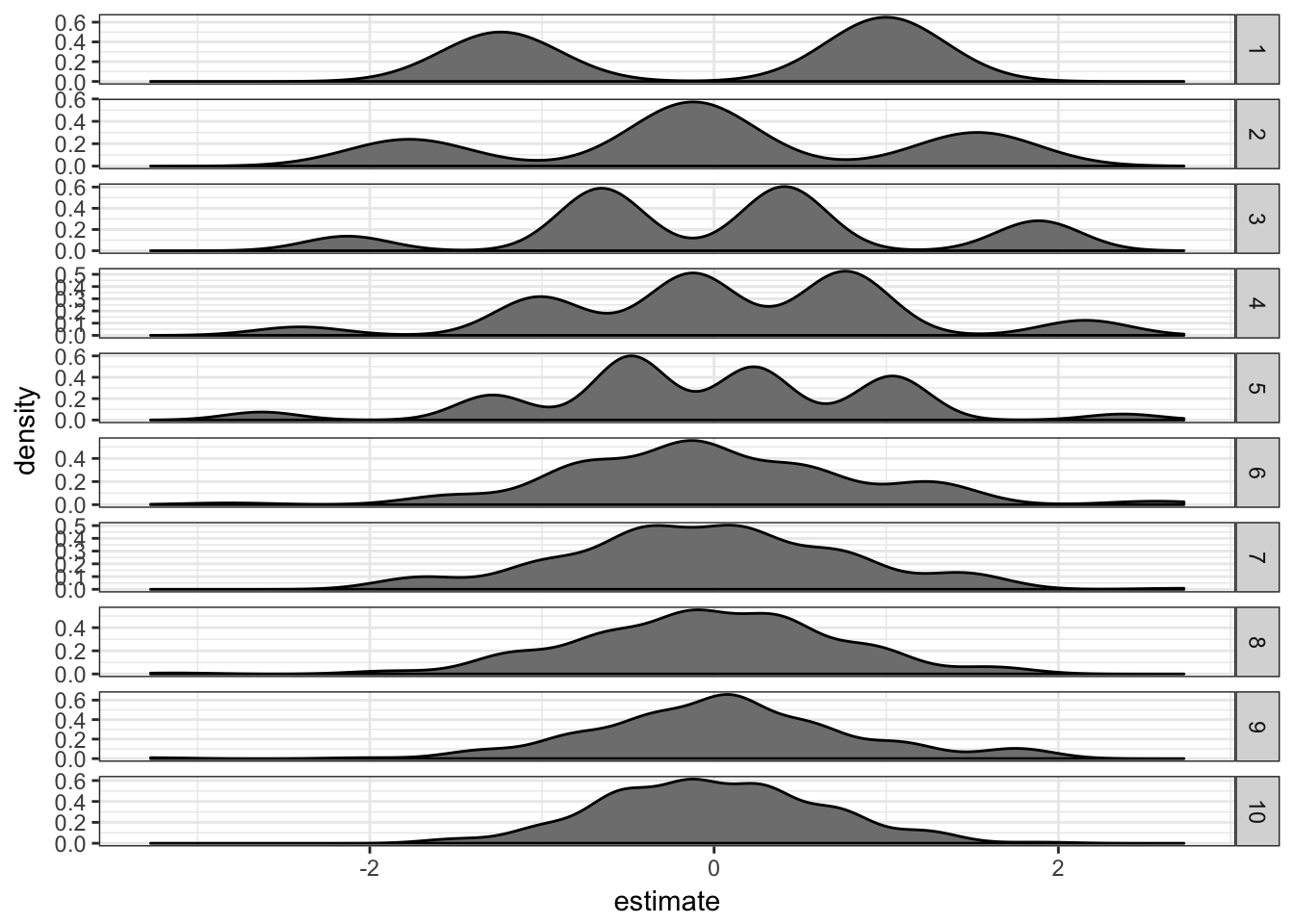This works well too.

A dummy example with some code for Generalized Additive Models, LOESS and SVM.

nb.samp = 1000
df = data.frame(x = runif(nb.samp, 0, 100))
df$y = rnorm(nb.samp, 0, 5) + abs(df$x - 25)
df$y = ifelse(df$x > 40, rnorm(nb.samp, 0, 5) - df$x * df$x/300 + 20, df\$y)
ggplot(df, aes(x = x, y = y)) + geom_point(alpha = 0.5) +
theme_bw()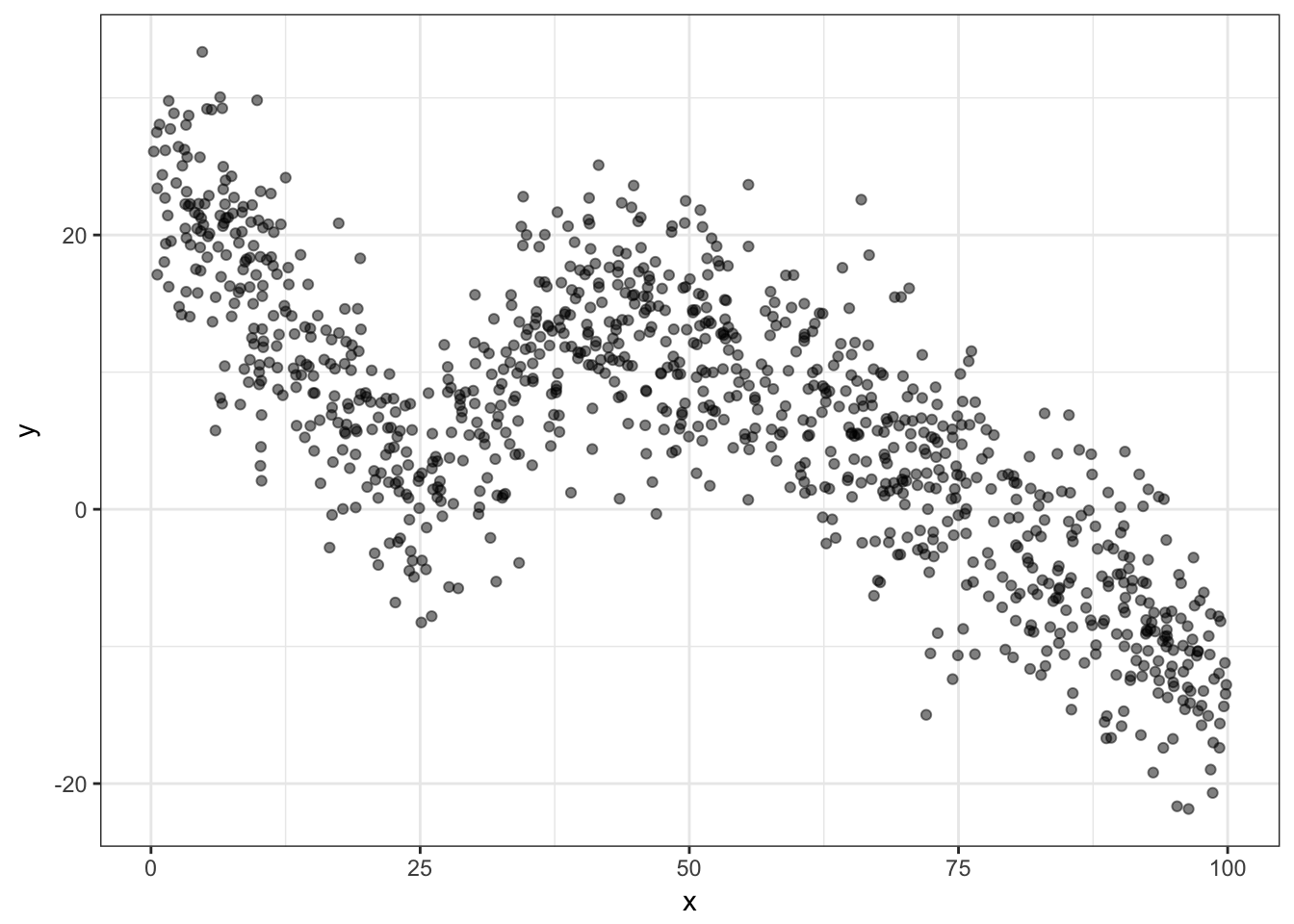glm.o = glm(y ~ x, data = df)
loess.o = loess(y ~ x, data = df)
library(mgcv)
gam.o = gam(y ~ s(x, bs = "cs"), data = df)
library(e1071)
svm.o = svm(y ~ x, data = df)

pred.df = rbind(df %>% mutate(y = predict(glm.o), model = "glm"),
df %>% mutate(y = predict(gam.o), model = "gam"),
df %>% mutate(y = predict(loess.o), model = "LOESS"),
df %>% mutate(y = predict(svm.o), model = "SVM"))

ggplot(df, aes(x = x, y = y)) + geom_point(alpha = 0.2) +
geom_line(aes(colour = model), size = 2, alpha = 0.9,
data = pred.df) + theme_bw() + scale_colour_brewer(palette = "Set1")